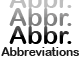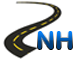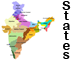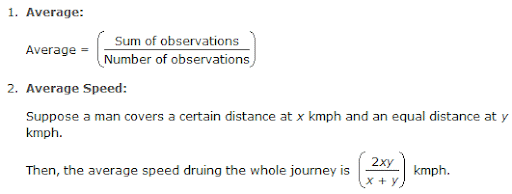Average -List of AbbreviationsNational Highways in IndiaStates & Union Territories of IndiaCurrency of All Countries

# Average

Overview:Sample Questions:

1. The average of first five multiples of 3 is :
A. 6 B. 9
C. 7.5 D. 12

Ans: B

2. A student was asked to find the arithmetic mean of the numbers 3, 11, 7, 9, 15, 13, 8, 19, 17, 21, 14 and x. He found the mean to be 12. What should be the number in the place of x ?
A. 3 B. 7
C. 17 D. 31

Ans: B

3. A library has an average of 510 visitors on Sundays and 240 on other days. The average number of visitors per day in month of 30 days beginning with a Sunday is:
A. 250 B. 276
C. 280 D.285

Ans: D

4. The average of 50 numbers is 30. If two numbers, 35 and 40 are discarded, then the average of the remaining numbers is nearly:
A. 28.32 B. 29.68
C. 28.78 D. 29.27

Ans: B

5. The average score of a cricketer for ten matches is 38.9 runs. If the average for the first six matches is 42, then find the average for the last four matches.
A. 33.25 B. 33.5
C. 34.25 D. 35

Ans: C

6. The average price of 10 books is Rs.12 while the average price of 8 of these books is Rs.11.75. Of the remaining two books, if the price of one book is 60% more than the price of the other, what is the price of each of these two books?
A. Rs.5, Rs.7.50 B. Rs.8, Rs.12
C. Rs.10, Rs.16 D. Rs.12, Rs.14

Ans: C

7. Of the three numbers, the first is twice the second and the second is twice the thirD. The average of the reciprocal of the numbers is . The numbers are:
A. 16, 8, 4 B. 20, 10, 5
C. 24, 12, 6 D. 36, 18, 9

Ans: C

8. Average of ten positive numbers is . If each number by 10%, then :
A. remains unchanged B. may decrease
C. may increase D. is increased by 10%

Ans: D

9. The average weight of three boys A, B and C is kg, while the average weight of three boys B, D and E is 53 kg. What is the average weight of A, B, C, D and E?
A. 52.4 kg B. 53.2 kg
C. 53.8 kg D. Data inadequate

Ans: D

10. The average age of 36 students in a group is 14 years. When teacher’s age is included to it, the average increases by one. What is the teacher’s age in years?
A. 31 B. 36
C. 51 D. None of these

Ans: C

11. A cricketer has a certain average for 10 innings. In the eleventh inning, he scored 108 runs, there by increasing his average by 6 runs. His new average is:
A. 48 runs B. 52 runs
C. 55 runs D. 60 runs

Ans: A

12. 10 years ago, the average age of a family of 4 members was 24 years. Tow children having been born (with age difference of 2 years), the present average age of the family is the same. The present age of the youngest child is:
A. 1 year B. 2 years
C. 3 years D. 5 years

Ans: C

13. The average salary of all the workers in a workshop is Rs.8000. The average salary of 7 technicians is Rs.12000 and the average salary of the rest is Rs.6000. The total number of workers in the workshop is:
A. 20 B. 21
C. 22 D. 23

Ans: B

14. In an examination, a pupil’s average marks were 63 per paper. If he had obtained 20 more marks for his geography paper and 2 more marks for his History paper, his average per paper would have been 65. How many papers was therein the examination?
A. 10 B. 11
C. 16 D. 12

Ans: B

15. The average age of students of a class is 15.8 years. The average age of boys in the class is 16.4 years and that of the girls is 15.4 years. The ratio of the number of boys to the number of girls in the class is:
A. 1 : 2 B. 2 : 3
C. 3 : 4 D. 3 : 5

Ans: B

16. What is the average salary of 15 employees?
I. Average salary of 7 clerical cadre (out of the 15 employees) is Rs.8500.
II. Average salary of 5 officer cadre (out of the 15 employees) is Rs.10000.
III. Average salary of the 3 sub-staff employees (out of 15 employees) is Rs.2500.

A. None B. I only
C. II only D. III only

Ans: A

17. The average of all odd numbers up to 100 is:
A. 50 B. 51
C. 62 D. 43

Ans: A

18. The average of 7 consecutive numbers is 33. The largest of these number is:
A. 30 B. 32
C. 38 D. 36

Ans: D

19. The average of four consecutive even numbers is 27. The largest of these number is:
A. 30 B. 31
C. 32 D. 33

Ans: A

20. The average height of 30 boys out of a class of 50, is 160cm, if the average height of the remaining boys is 165cm, the average height of the whole class (in cm) is:
A. 120 B. 151
C. 162 D. 163

Ans: C

21. The average of tow numbers is M. If one is N, then the other number is:
A. 2N-M B. 2M-N
C. 2N-2M D. 2M-2N

Ans: B

22. The average of 5 results is 46 and that of the first 4 is 45. The fifth result is:
A. 50 B. 61
C. 52 D. 43

Ans: A

23. The average of three numbers is 20. If two numbers are 16 and 22 the third number is:
A. 20 B. 21
C. 22 D. 23

Ans: C

24. The average of eight numbers is 14. The average of six of these numbers is 16. The average of remaining two numbers is :
A. 10 B. 11
C. 12 D. 8

Ans: D

25. The average of ten numbers is 7. If each number is multiplied by 12, then the average of new set of numbers is:
A. 80 B. 81
C. 82 D. 84

Ans: DClick Here to Find Latest Jobs and Current Affairs

Updated: May 31, 2013 — 10:48 am